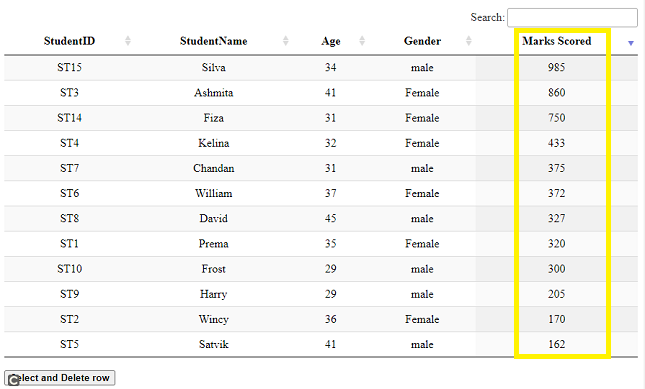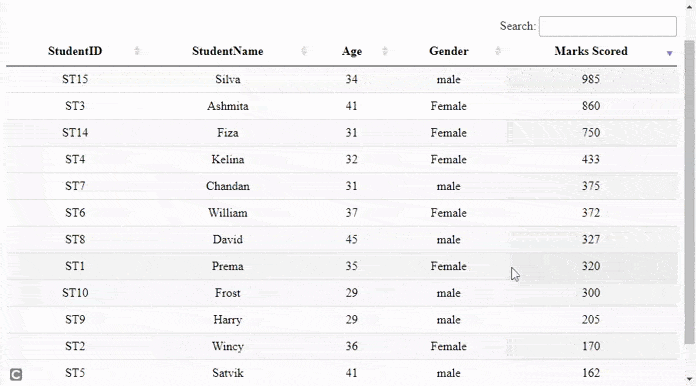Related Articles

# How to implement single row select and delete using DataTables plugin ?

• Last Updated : 31 May, 2021

DataTables are a modern jQuery plugin for adding interactive and advanced controls to HTML tables for a webpage. It is a very simple-to-use plug-in with a variety of options for the developer’s custom changes as per the application’s need. The plugin’s features include pagination, sorting, searching, and multiple-column ordering.

In this article, we will demonstrate the implementation of single row deletion after selecting the row using the DataTables plugin.

Approach: In the following example, DataTables uses the student details from the HTML table as the main source. Each row in the table shows details for one student’s information.

• A DataTable is initialized.
• The developer can set the features of paging or searching as per the need as shown in the script part of the code.
• The table is set in the descending order of student’s marks just to show the ordering usage.
• Check if the row selected by the user has class “selected” and then remove it.
• If the class is not “selected” then it is removed from all other rows in the table and the class is added to the row being selected.
• On button click, remove the row along with setting the draw() API to false.
• The draw() API is used to reflect the changes after an action in the table.

The pre-compiled files which are needed to implement are

CSS:

`https://cdn.datatables.net/1.10.22/css/jquery.dataTables.min.css`

JavaScript:

```https://code.jquery.com/jquery-3.5.1.js
https://cdn.datatables.net/1.10.22/js/jquery.dataTables.min.js```

Example: The following example demonstrates the above approach showing single row select and then delete the row.

## HTML

 ```<``html``>`` ` `<``head``>`` ` `    ``<``meta` `content="``initial-scale``=``1``, ``maximum-scale``=``1``,``                   ``user-scalable``=``0``" ``name``=``"viewport"` `/>``    ``<``meta` `name``=``"viewport"` `content``=``"width=device-width"` `/>`` ` `    ````    ``<``link` `rel``=``"stylesheet"` `href``=``"https://cdn.datatables.net/1.10.22/css/jquery.dataTables.min.css"` `/>`` ` `    ````    ``<``script` `type``=``"text/javascript"` `        ``src``=``"https://code.jquery.com/jquery-3.5.1.js"``>``    ```` ` `    ````    ``<``script` `type``=``"text/javascript"` `src``=``"https://cdn.datatables.net/1.10.22/js/jquery.dataTables.min.js"``>``    ```` ` `    ``<``style``>``        ``td {``            ``text-align: center;``        ``}``    `````` ` `<``body``>``    ``<``h2``>Delete single row using DataTables plugin`` ` `    ````    ``<``table` `id``=``"tableID"` `class``=``"display"` `style``=``"width:100%"``>``        ``<``thead``>``            ``<``tr``>``                ``<``th``>StudentID``                ``<``th``>StudentName``                ``<``th``>Age``                ``<``th``>Gender``                ``<``th``>Marks Scored``            ````        ```` ` `        ``<``tbody``>``            ``<``tr``>``                ``<``td``>ST1``                ``<``td``>Prema``                ``<``td``>35``                ``<``td``>Female``                ``<``td``>320``            ````            ``<``tr``>``                ``<``td``>ST2``                ``<``td``>Wincy``                ``<``td``>36``                ``<``td``>Female``                ``<``td``>170``            ````            ``<``tr``>``                ``<``td``>ST3``                ``<``td``>Ashmita`` ` `                ``<``td``>41``                ``<``td``>Female``                ``<``td``>860``            ````            ``<``tr``>``                ``<``td``>ST4``                ``<``td``>Kelina``                ``<``td``>32``                ``<``td``>Female``                ``<``td``>433``            ````            ``<``tr``>``                ``<``td``>ST5``                ``<``td``>Satvik``                ``<``td``>41``                ``<``td``>male``                ``<``td``>162``            ````            ``<``tr``>``                ``<``td``>ST6``                ``<``td``>William``                ``<``td``>37``                ``<``td``>Female``                ``<``td``>372``            ````            ``<``tr``>``                ``<``td``>ST7``                ``<``td``>Chandan``                ``<``td``>31``                ``<``td``>male``                ``<``td``>375``            ````            ``<``tr``>``                ``<``td``>ST8``                ``<``td``>David``                ``<``td``>45``                ``<``td``>male``                ``<``td``>327``            ````            ``<``tr``>``                ``<``td``>ST9``                ``<``td``>Harry``                ``<``td``>29``                ``<``td``>male``                ``<``td``>205``            ````            ``<``tr``>``                ``<``td``>ST10``                ``<``td``>Frost``                ``<``td``>29``                ``<``td``>male``                ``<``td``>300``            ```` ` `            ``<``tr``>``                ``<``td``>ST14``                ``<``td``>Fiza``                ``<``td``>31``                ``<``td``>Female``                ``<``td``>750``            ````            ``<``tr``>``                ``<``td``>ST15``                ``<``td``>Silva``                ``<``td``>34``                ``<``td``>male``                ``<``td``>985``            ```` ` `        ````    ````    ``<``br` `/>`` ` `    ``<``button` `id``=``"btnID"``><``b``>Select and Delete row`` ` `    ``<``script``>`` ` `            ``/* Initialization of datatables */``            ``\$(document).ready(function () {`` ` `                ``// Paging and other information are``                ``// disabled if required, set to true``                ``var mytableVar = \$('#tableID').DataTable({``                    ``"paging": false,``                    ``"info": false``                ``});`` ` `                ``// Ordering the table in descending``                ``// order of students marks``                ``mytableVar.order([[4, 'desc']]).draw(false);`` ` ` ` `                ``\$('#tableID tbody').on('click', 'tr', function () {`` ` `                    ``// Check for 'selected' class and remove``                    ``if (\$(this).hasClass('selected')) {``                        ``\$(this).removeClass('selected');``                    ``}``                    ``else {``                        ``mytableVar.\$('tr.selected').removeClass('selected');``                        ``\$(this).addClass('selected');``                    ``}``                ``});`` ` `                ``// On button click, remove the row with setting``                ``// the draw() to false``                ``// draw() API is used to reflect the changes``                ``// after a action``                ``\$('#btnID').click(function () {``                    ``mytableVar.row('.selected').remove().draw(false);``                ``});``            ``});``    `````` ` ``

Output:

• Before Clicking the button: Notice that the marks scored by the students in the last column are in descending order.• After Clicking the button: The following output shows that the row for student name “William” is deleted.Attention reader! Don’t stop learning now. Get hold of all the important HTML concepts with the Web Design for Beginners | HTML  course.

My Personal Notes arrow_drop_up